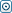Forex Stock Exchange Forum
Would you like to react to this message? Create an account in a few clicks or log in to continue.

# Simple way to get data from yahoo financegandra
Global Moderator
Number of messages : 3651
Points : 9321
Date of Entry : 2013-01-13
Year : 50
Residence Country : Serbia##Simple way to get data from yahoo finance

on Wed Mar 13, 2019 10:42 pm
Hi guys,
If you are passionate stock market trader, and love to have your own japanese candlestick chart for technical analysis, and not know how to do this. I will show you most comon way, in a few simple steps. This  example show you how to get "Google" stock data from yahoo finance.  Code is written in Python .

So, let's get startedThe first file : "yahoo_finance_data.py",  allows you to get financial data from yahoo for preferred stock , and store them in one csv file.
Code:
`# import matplotlib.pyplot as plt# from matplotlib import styleimport pandas as pdimport datetime as dt     import pandas_datareader.data as pdtr       #style.use('ggplot')start_date = dt.datetime(2010, 1, 1)end_date =  dt.datetime.now()df = pdtr.DataReader('GOOG', 'yahoo', start_date, end_date)#print(df)#print(df.head())#print(df.tail())df.to_csv('goog.csv')`

Now when we have information about Google price movement for the desired time interval, we need to switch them to the chart. Make new python  file : " plot_linear_data.py " , and write this code below.
Code:
`import matplotlib.pyplot as pltfrom matplotlib import styleimport pandas as pdimport datetime as dtimport pandas_datareader.data as pdtrstyle.use('ggplot')df = pd.read_csv('goog.csv', parse_dates=True, index_col=0)#print(df.head())# print(df[['Open', 'High']])# df['Adj Close'].plot()# plt.show()df['100ma'] = df['Adj Close'].rolling(window=100, min_periods=0).mean()df.dropna(inplace=True)print(df.head())ax1 = plt.subplot2grid((6, 1), (0, 0), rowspan=5, colspan=1)ax2 = plt.subplot2grid((6, 1), (5, 0), rowspan=1, colspan=1, sharex=ax1)ax1.plot(df.index, df['Adj Close'])ax1.plot(df.index, df['100ma'])ax2.bar(df.index, df['Volume'])plt.show()`

and  result is a nice linear chart:
[You must be registered and logged in to see this image.]

Next step is candlestick chart. So , make new python file:" candlestick_graf.py ",  and copy/paste code below.
Code:
`import matplotlib.pyplot as pltfrom matplotlib import stylefrom mpl_finance import candlestick_ohlcimport matplotlib.dates as mdatesimport pandas as pdimport datetime as dtimport pandas_datareader.data as pdtrstyle.use('ggplot')df = pd.read_csv('goog.csv', parse_dates=True, index_col=0)# df['100ma'] = df['Adj Close'].rolling(window=100, min_periods=0).mean()df_ohlc = df['Adj Close'].resample('10D').ohlc()df_volume = df['Volume'].resample('10D').sum()df_ohlc.reset_index(inplace=True)df_ohlc['Date'] = df_ohlc['Date'].map(mdates.date2num)ax1 = plt.subplot2grid((6, 1), (0, 0), rowspan=5, colspan=1)ax2 = plt.subplot2grid((6, 1), (5, 0), rowspan=1, colspan=1, sharex=ax1)ax1.xaxis_date()candlestick_ohlc(ax1, df_ohlc.values, width=2, colorup='g')ax2.fill_between(df_volume.index.map(mdates.date2num), df_volume.values, 0)plt.show()`

And vuala we have nice candlestick graph in the range of ten days per each candle.

[You must be registered and logged in to see this image.]

[You must be registered and logged in to see this image.]
Permissions in this forum:
You cannot reply to topics in this forum